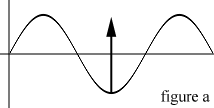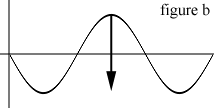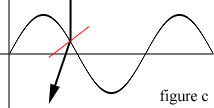2019/01/07 16:43

# 概述：

## 第 1 部分 - 如何模拟波

### b. 区域采样案例：模糊效果

ResultMap[x, y] := ( SourceMap[x, y] +
SourceMap[x+1, y] +
SourceMap[x-1, y] +
SourceMap[x, y+1] +
SourceMap[x, y-1] ) DIV 5


(x, y) 值取决于周围值的平均值，当然图片模糊算法没那么简单，不过思路大致如此。

ResultMap[x, y] := (( CurrentSourceMap[x+1, y] +
CurrentSourceMap[x-1, y] +
CurrentSourceMap[x, y+1] +
CurrentSourceMap[x, y-1] ) DIV 2 ) -
PreviousResultMap[x, y]### d. 波形模拟示例

.
.
const
MAXX = 320; { 贴图的宽高 }
MAXY = 240;
DAMP = 16; { 阻尼系数 }

{ 定义 WaveMap[frame, x, y] 和 frame-indices }
var
WaveMap: Array[0..1, 0..(MAXX-1), 0..(MAXY-1)] of SmallInt;
CT, NW: SmallInt;

.
.
procedure UpdateWaveMap;
var
x,y,n: Smallint;
begin
{ 跳过边界用以区域采样 }
for y := 1 to MAXY-1 do begin
for x := 1 to MAXX-1 do begin
n := ( WaveMap[CT,x-1,y] +
WaveMap[CT,x+1,y] +
WaveMap[CT,x,y-1] +
WaveMap[CT,x,y+1] ) div 2 -
WaveMap[NW,x,y];

n := n - (n div DAMP);

WaveMap[NW,x,y] := n;
end;
end;
end;
.
.


Temporary_Value := CT;
CT := NW;
NW := Temporary_Value;


### e. 开始运动

WaveMap[x, y] := -100;


## 第2部分-透明表面光线追踪### a. 确定入射光的角度[bquote]折射率= sin（入射光的角度）/ sin（折射光的角度）[/ bquote]，

[bquote]折射光的角度= arcsin（sin （入射光的角度）/折射率）[/ bquote]
，其中折射率是水的折射率：2.0。

[bquote]位移= tan（折射光束的角度）*高度差[/ bquote]

for y := 1 to MAXY-1 do begin
for x := 1 to MAXX-1 do begin
xDiff := Trunc(WaveMap[x+1, y] - WaveMap[x, y]);
yDiff := Trunc(WaveMap[x, y+1] - WaveMap[x, y]);

xAngle := arctan( xDiff );
xRefraction := arcsin( sin( xAngle ) / rIndex );
xDisplace := Trunc( tan( xRefraction ) * xDiff );

yAngle := arctan( yDiff );
yRefraction := arcsin( sin( yAngle ) / rIndex );
yDisplace := Trunc( tan( yRefraction ) * yDiff );

if xDiff < 0 then begin
{ Current position is higher - Clockwise rotation }
if yDiff < 0 then
newcolor := BackgroundImage[x-xDisplace, y-yDisplace]
else
newcolor := BackgroundImage[x-xDisplace, y+yDisplace]
end else begin
{ Current position is lower - Counterclockwise rotation }
if yDiff < 0 then
newcolor := BackgroundImage[x+xDisplace, y-yDisplace]
else
newcolor := BackgroundImage[x+xDisplace, y+yDisplace]
end;

TargetImage[x, y] := newcolor;
end;
end;


ps：文章是千禧年，所以别吐槽为啥作者用Pascal编写了，问就是 聪明的程序员 (σ°∀°)σ..:*☆ ，大家就当伪代码看好了~

0
0 收藏

### 作者的其它热门文章0 评论
0 收藏
0